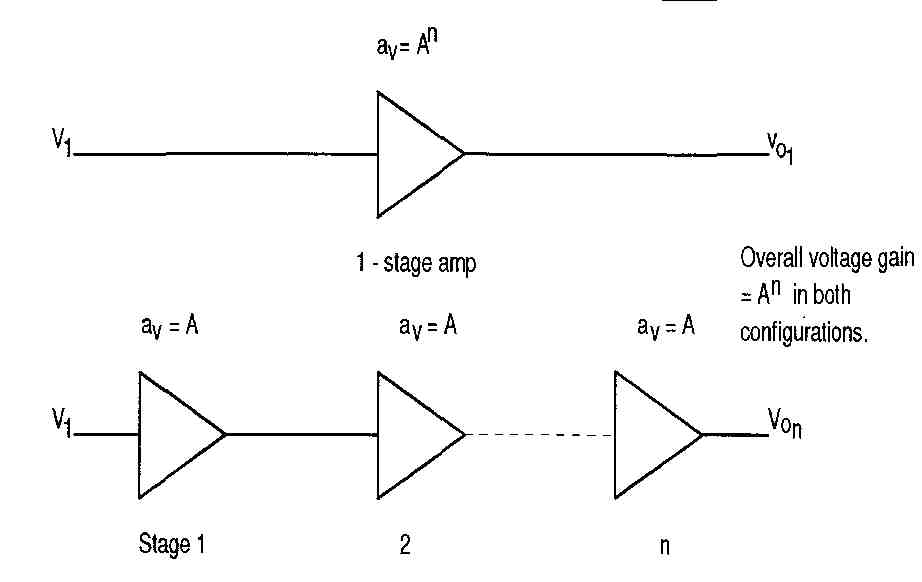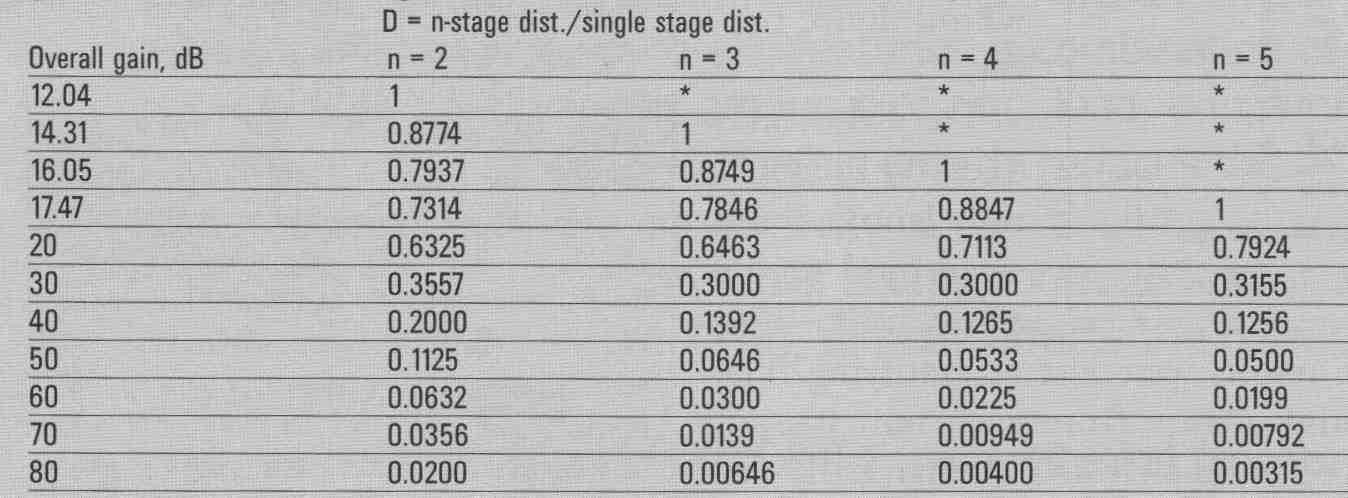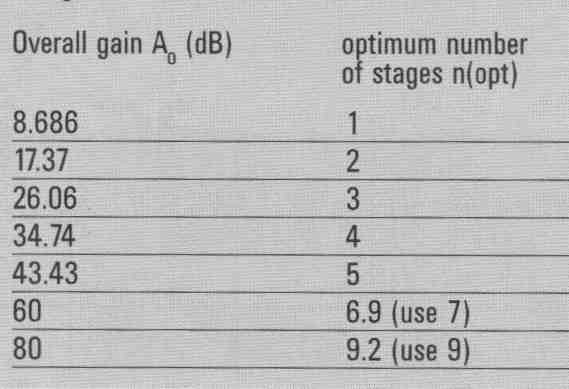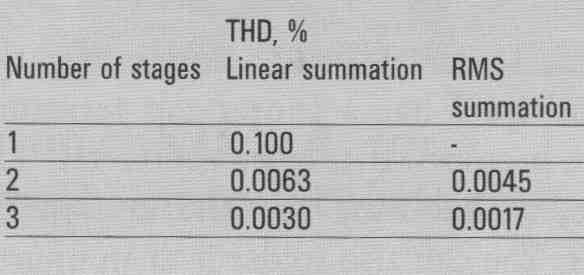# Optimum Stages for Minimal Distortion

The optimum number of cascaded equal-gain op-amp-type stages is mathematically derived for a given required overall gain, to produce the lowest distortion.

A two-stage amplifier has lower distortion than a single-stage amp if the required over 11 gain is greater than 4 (12.04dB); for a three-stage amp the “benefit point” overall gain (BPG) is 3 _/3 (14.31dB). For “n” stages, BPG = n(n/n-1) BPG (dB) = [20n/n-1] log n. With greater gain, the n-stage has less distortion.

PERSPECTIVE

It’s often assumed that the fewer number of gain stages, the better. This is often not true. This article focuses on negative feedback (NFB) stages such as op amps, but even with open-loop circuits such as triode stages, more stages can be better. A good example occurs when a 2 triode stage cancels most of the 1st stage’s nonlinearity.

By “op amp-type” I mean any amplifier of high open-loop gain that is greatly (and with high stability) reduced by NFB. The distortion/signal ratio is also greatly reduced, in proportion to the gain reduction, and without introducing audible “mystery artifacts” (e.g., phase- modulated slewing AM to FM conversion, and so on) if the overall loop has a high stability and headroom margin, not involving complex components such as load-sensitive transformers.

SOMETIMES MORE IS BETTER

To intuitively understand the reason for multi-stage distortion reduction, consider a single-stage versus a two-stage design, both having 40dB (x 100) over all gain, and using the AD797 op amp. Its unity-gain THD is 0.0001% (one part per million, or 1 x 10^-6). The single-stage amp would have a closed-loop (CL) gain of 100, so the THD is 100 times the unity-gain level (1/100 the NFB); that is, 0.01%, or 1 x 10^-4.

Meanwhile, each stage of the two- stage amp needs a CL gain of only 10 (20dB), so its THD is 0.001%. Now, with such (typical of good op amps) low fractional distortion levels (1 x 10 here), cascading stages can at most linearly sum the individual stage THD levels. With the 90° phase shift associated with the first-order (6dB/octave) slopes, such as in RIAA equalizers, the individual stages’ distortion products will RMS sum, resulting in lower overall distortion.

But back to the linear addition in flat- response amps, the two-stage design then has an overall THD of 0.002%, which is only one-fifth that of the single-stage amp! This is because in the multi-stage amp the gains multiply, but the distortion/signal ratios only add (at most). Another benefit is that the band width is higher.

This is not a free lunch, but the price is only another op amp.

Some of you may object to this setup, asking, “But the signal is going through twice as many amplifying devices—isn’t that worse?”

COUNTER ARGUMENT 1

From the microphones to the disc (vinyl or CD, SACD, and so on), the signal has already traveled through many amplifying devices, most of which are not considered audiophile quality. Yet somehow many recordings still sound very good.

The resolution—”sandpapering” of the insufficient 16 bits on a CD—is a much worse degradation than that produced (if any) by a high-quality small- signal Class A operated transistor or tube. So why worry about a (relatively) very few more amplifying devices?

For those who believe that having the fewest possible components is always best, I suggest listening to an Edison horn Victrola! “All generalities are false, including this one.”

COUNTER ARGUMENT 2

The signal degradation of a device or combination has a name: distortion. The overall distortion is what matters. Now, I realize that THD numbers often don’t relate to sound quality, for a number of good reasons:

1. Low-level high-order distortion is worse than vice versa, within reason able maximum levels of low-order distortion.

2. THD numbers might not show “hidden” distortion, such as slew-rate limiting that acts only on fast peaks, per haps with ultrasonic content.

3. Reactive loads are usually not included in power amp testing.

4. Quantizing/time-based jitter in digital processing may escape measurement detection, but that’s the fault of present techniques, which don’t use complex “real-world” signals involving the full dynamic range.

None of the above applies with well-de signed and utilized small-signal Class A operated components considered in this article, such as high-quality op-amps supplying load currents small enough to keep their output stages in Class A.

ANALYSIS

This is based on three assumptions that are closely realized in high-quality op amps:

1. Closed-loop distortion (d) is directly proportional to closed-loop gain. This is because the NFB reduces both gain and the distortion/signal ratio.

2. Each stage’s distortion is a very small fraction of 1, with regard to the signal. For example, the AD797 THD in unity gain is 0.0001%, so d = 10^-6. With 40dB closed-loop gain, THD is 0.01% (d = 10^-4)

3. With n cascaded stages, d is multi plied by n. That is, the “d” terms add linearly. For d CC1, this is very close. This is a worst-case addition, as previously discussed.

Table 2 summarizes some results. It shows that in a multi-stage amp, the ideal gain per stage (for lowest output distortion) is 8.686dB. This is a voltage gain of e = 2.718, which is the base of natural logarithms. A natural result!FIGURE 1: Single and multi-stage amplifiers.

DISCUSSION

Contrary to possibly intuitive expectations, partitioning the required overall gain of an amplifier into multiple cascaded equal-gain stages will produce lower distortion than with all the gain in a single stage, if the gain stages are of op amp topology; that is, with high open-loop gain and negative feedback; and if the required overall gain exceeds 4 (12.04dB).

PRACTICAL EXAMPLE

Consider a 60dB amplifier of flat frequency response (as discussed, this produces worst-case linear addition of multiple stages’ distortion terms). With ex tended frequency response slopes, such as with RIAA equalization, those terms will approximate RMS addition, or possibly even cancellation.

1. A two-stage amp has 0.0632 (maximum) times the THD of a single stage with the same 60dB overall gain.

2. A three-stage amp has 0.0300 (maximum) times the single-stage THD.

3. The three-stage has 0.475 times the two-stage THD.

For a specific example, consider a 60dB amplifier using AD797 op amps, unity-gain THD of 0.0001% (Table 3).

BANDWIDTH OPTIMIZING

The optimum gain per stage is 4.343dB, exactly half that (in dB) for minimal distortion.TABLE 1: Distortion reduction factor re single-stage amplifier, function of overall gain and number of stages.TABLE 2: Optimum number of stages for lowest distortion, function of over all gain in dB.TABLE 3: Example of 60dB amplifier

============

Updated: Sunday, 2018-05-06 7:44 PST